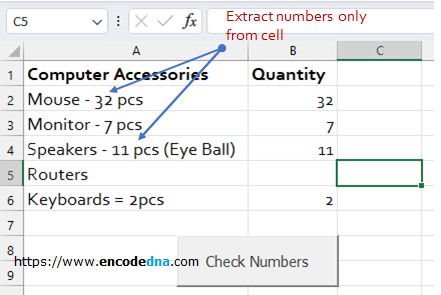﻿ How to check if a Cell in an Excel Worksheet has numbers using VBA

# How to check if a Cell in an Excel Worksheet has numbers using VBA

Excel VBA provides many built-in functions, which helps programmers accomplish a task. I am creating a simple scenario here to use one of the built-in functions called IsNumeric() to check if a particular cell in my Excel Worksheet has numbers.

## IsNumeric() Syntax

IsNumeric( expression )

The IsNumeric() function (like Excel ISNUMBER()) returns a boolean true or false. It takes a parameter, a variant value for evaluation.

A cell in an Excel worksheet can have a variety of data in the form of text, numbers, formulas etc. If you are a VBA programmer, you can simply use the function "IsNumeric()" to check if the cell has a number. However, a cell may have alphanumeric values too and if that is the case, then I want to find (or extract) only the numbers from string and show it in another cell. Please see the image below.The VBA Code

Press Alt+F11 to open VBA and click Project Explorer from the tool bar. In the Project Explorer window, expand Microsoft Excel Object and double click Sheet1. That’s where I have my data. Now write the below code.

```Option Explicit

Private Sub CommandButton1_Click()
Worksheets("Sheet1").Range("B2:B10").ClearContents      ' CLEAR ALL THE VALUES.
checkNumber (Worksheets("Sheet1").Range("A2:A10"))
End Sub

Sub checkNumber(objRange As Range)

Dim myAccessary As Variant
Dim i As Long
Dim iRow As Long

iRow = 2

For Each myAccessary In objRange  ' Loop through values in each row.
For i = 1 To Len(myAccessary.value) ' Loop through each character of teh string (value in the cell).

If IsNumeric(Mid(myAccessary.value, i, 1)) Then    ' Now, check if character is a number.
' If its a number, show the number in the 2nd column.
If Trim(objRange.Cells(objRange.Row - 1, 2)) <> "" Then
objRange.Cells(iRow - 1, 2) = _
objRange.Cells(iRow - 1, 2) & Mid(myAccessary.Text, i, 1)
Else
objRange.Cells(iRow - 1, 2) = Mid(myAccessary.Text, i, 1)
End If
End If

Next i
iRow = iRow + 1
Next myAccessary
End Sub```

Alternatively, you can use RegEx to check if a cell has numbers.

Check this out

Let me explain the above macro.

It calls a function named checkNumber() upon button click. The procedure takes a parameter in the form of a Range. Therefore, I am passing the Range ("A2:A10"), where I have my data with cells that might have numbers.

I have two For loops. The first loop gets the element (cell values in every Row) and the second loop extracts each character in the cell values, using the function Mid().

Mid(myAccessary.value, i, 1)

Next, I have a condition that checks if the character extracted using the function "Mid()" is a number or not. The IsNumeric() function evaluates the character. When it finds the number, it writes it in the next cell (Column Quantity) of the same row. If there are more than one numbers in the cell, I’ll concatenate the values and show it.

In this way I’ll check and extract the numbers from a given string and get the Quantity in Pcs.

You may also like this: How to Find and Highlight Duplicate Values in Excel using VBA

Well, that’s it. Thanks for reading.